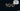# Move Zeroes - Leet Code Solution

September 03, 2020## Problem Statement

Given an array nums, write a function to move all 0’s to the end of it while maintaining the relative order of the non-zero elements.

Example

``````Input: [0,1,0,3,12]
Output: [1,3,12,0,0]``````

## Solution (copying to left)

We can keep a index variable which will keep a tab on all non-zero values.

On iteration, we can move each non-zero value to left side.

### Code

``````public void moveZeroes_simple(int[] nums) {
int left=0;

for (int i=0; i<nums.length; i++) {
if (nums[i] != 0) {
nums[left] = nums[i];
left ++;
}
}

//copy zeroes to remaining array
for (int i=left; i<nums.length; i++) {
nums[i] = 0;
}
}``````

### Complexity

Its `O(n)`

## Another Solution (Swapping)

We can do a slight modification to above solution. The point where we just move non-zero value to left. We can do a swap as well.

### Code

``````public void moveZeroes(int[] nums) {
int left=0;

for (int i=0; i<nums.length; i++) {
if (nums[i] != 0) {
//swap
int t = nums[i];
nums[i] = nums[left];
nums[left] = t;

left ++;
}
}
}``````

### Complexity

Its `O(n)`
But, its better since we are not using another loop to copy zero.